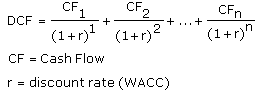### Would you like to switch to our mobile app?

Chapter 23 — Valuation: Discounted Cash Flow
Font type: Sans-Serif
Font size: Large

## Valuation: Discounted Cash Flow

After knowing what to look for in an investment, your ultimate goal is to find what the investment is worth. To be successful with fundamental analysis, you must put a number value on your potential investment or you won’t be able to compare price and value. This is probably the most difficult part about fundamental analysis, but it is very important because it tells you whether you should buy and gives a guideline for when you should sell.

There are two main ways to come up with valuation numbers for a business. The first is the Discounted Cash Flow (DCF) Valuation, which gives you an absolute numerical value for the investment. The second method is Ratio Valuation, which gives you a value based on comparing the stock of your company relative to the prices of similar investments. Ratio valuation is easier to do, but its results are less accurate.

A. Discounted Cash Flow (DCF) Valuation

The purpose of the Discounted Cash Flow model is to calculate what a company is a worth based on the amount of cash its business is generating. The basic way that this is done is by adding up the amount of cash that the company is expected to make over the next few years (for example, if your time period is 10 years).

But a dollar today is usually worth more than a dollar later, so each year’s cash flow must be “discounted” back to the present day, and then added in. The math behind this calculation can become complex, but using this model will give you the most rational and thoughtful valuation numbers. It also requires a thorough evaluation of the company’s potential profitability, so the more predictable the cash flows are, the easier it is to use DCF. The equation looks like this:i. Forecasting Growth

In order to determine the future cash flows, you must first decide how much growth the company will be able to achieve. This growth assumption is based mostly on the qualitative factors that you should have evaluated. If the company is growing faster than the overall economy, those qualitative factors will help you decide how long to assume the company will continue at such a fast rate of growth. For companies with strong competitive advantages, their period of above-average returns will be much longer. You will also want to take into account the company’s average growth rate for the past few years.Once you have decided on an investment time period and found the most likely length of fast growth, you can come up with two forecasts. One for optimal growth and one for more realistic growth. When you have done that, you can determine the amount of revenue that will be turned into free cash flow.

Forecast Revenue Growth Profilesii. Forecasting Free Cash Flow

The most basic way to calculate free cash flow is to start with cash flow from operations and subtract capital expenditures. What you will do next is find the percentage of revenue that becomes free cash flow. So for example, if the company’s revenue for the year is \$100 million, and its free cash flow is \$30 million, then the free cash flow is 30% of revenue.

Then you take this 30% figure and multiply the revenue for each year in your optimistic forecast by 30% (so you are assuming that profitability does not decrease). For the realistic forecast, you should decrease this profitability percentage as you decrease the growth rate (assuming profitability decreases over time). You will have two free cash flow forecasts; one optimistic and the other more realistic.

iii. Finding the Discount Rate

A commonly used discount rate is the weighted average of the company’s cost of capital (how much it “costs” to have their combination of debt and equity), but when we are working with a range of numbers it isn’t necessary to precisely calculate the discount rate.

In general, the better the company’s competitive advantage or the more debt it holds, the lower the discount rate you can use. This should range between 5% and 15%. For a very strong company, you can use the interest rate on U.S. Treasury Bonds, but when interest rates are low a good rule of thumb is 10%.

iv. Fair Value

Once you have calculated all of the numbers, then you will be ready to come up with your fair value estimate. In order to do this, you will have to get an estimate for the amount of cash flows that the company will produce after our forecast time period. The fastest way to do this is to take the cash flow from the last period and multiply it by some multiple.

The multiple that you use will have a large impact on the fair value that you find, so it is important not to be overly optimistic. You should pick a number based on the company’s average Price/Earnings for the past five years. If this is not realistic, use a number between 10 and 20 (lower numbers are far more conservative estimates).

After calculating all of these numbers you can find the total value of the company by adding up the cash flow for each time period and the “forever” cash flow numbers at the end.The number that you will find is the total value of the company, but since you are an equity investor, you want to know how much of that value is captured by equity investors. The final step is to subtract the total amount of debt that the company holds. That range is the equity value of the company. If the company’s shares are selling at a significant discount to this number, it would be considered a buying opportunity. If the shares are selling for a much higher value, then you would want to consider selling.